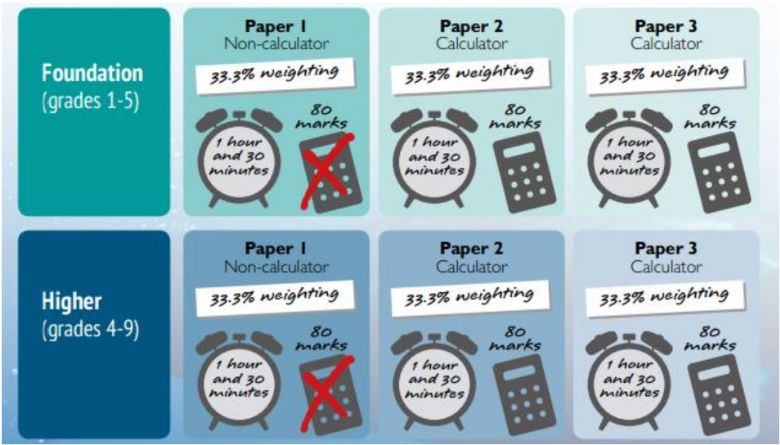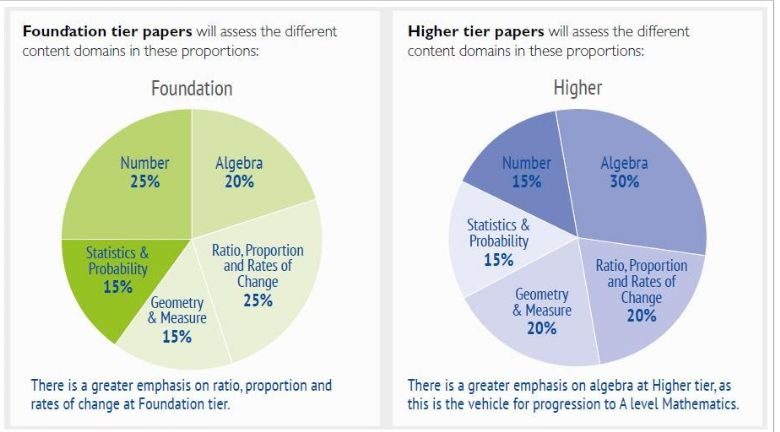Skip to content ↓# New Maths GCSE

Mathematics is an exciting new course that holds an emphasis on real life functional tasks and problem solving. Students will learn a range of concepts and get the opportunity to practice transferring these to solve real life problems. The course will develop student’s skills across the following areas. The grading has been changed from A*-G and now uses the new national 1-9 grading system.

The GCSE Course followed is Edexcel Level 1/Level 2 GCSE (9–1) in Mathematics (1MA1).  Here is a summary:The aims of the course are to enable pupils to:

• develop fluent knowledge, skills and understanding of mathematical methods and concepts
• acquire, select and apply mathematical techniques to solve problems
• reason mathematically, make deductions and inferences, and draw conclusions
• Comprehend, interpret and communicate mathematical information in a variety of forms appropriate to the information and context.### Important Formula